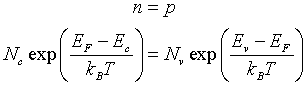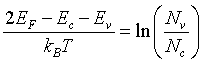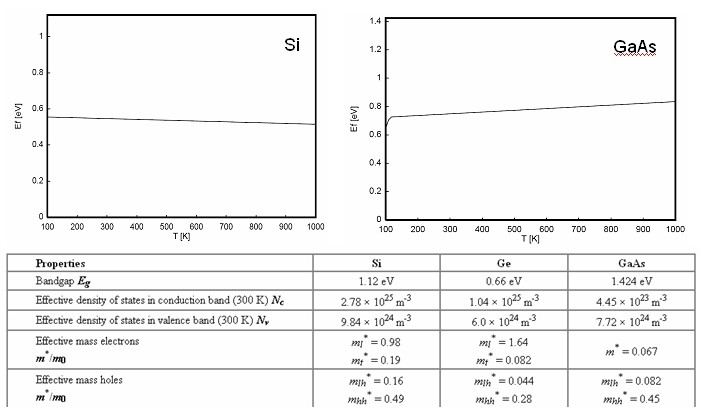PHT.301 Physics of Semiconductor Devices

## Fermi energy of an intrinsic semiconductor

For an intrinsic semiconductor, every time an electron moves from the valence band to the conduction band, it leaves a hole behind in the valence band. The density of electrons in the conduction band equals the density of holes in the valence band.Here Nc is the effective density of states in the conduction band, Nv is the effective density of states in the valence band, EF is the Fermi energy, Ec is the conduction band edge, Ev is the valence band edge, kB is Boltzmann's constant, and T is the temperature in K.

Rearranging this equation yields,Take the logarithm,Solve for EF,The Fermi energy is in the middle of the band gap (Ec + Ev)/2 plus a small correction that depends linearly on the temperature. The correction term is small at room temperature since Eg ~ 1 eV while kBT ~ 0.025 eV. For Si and Ge, Nc > Nv and the correction term is negative while for GaAs Nc < Nv and the correction term is positive.